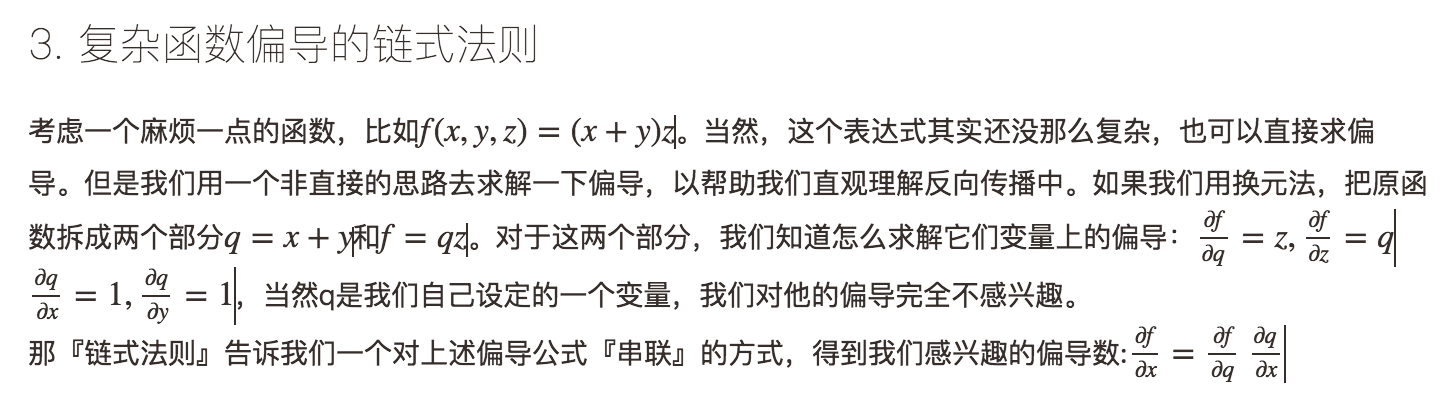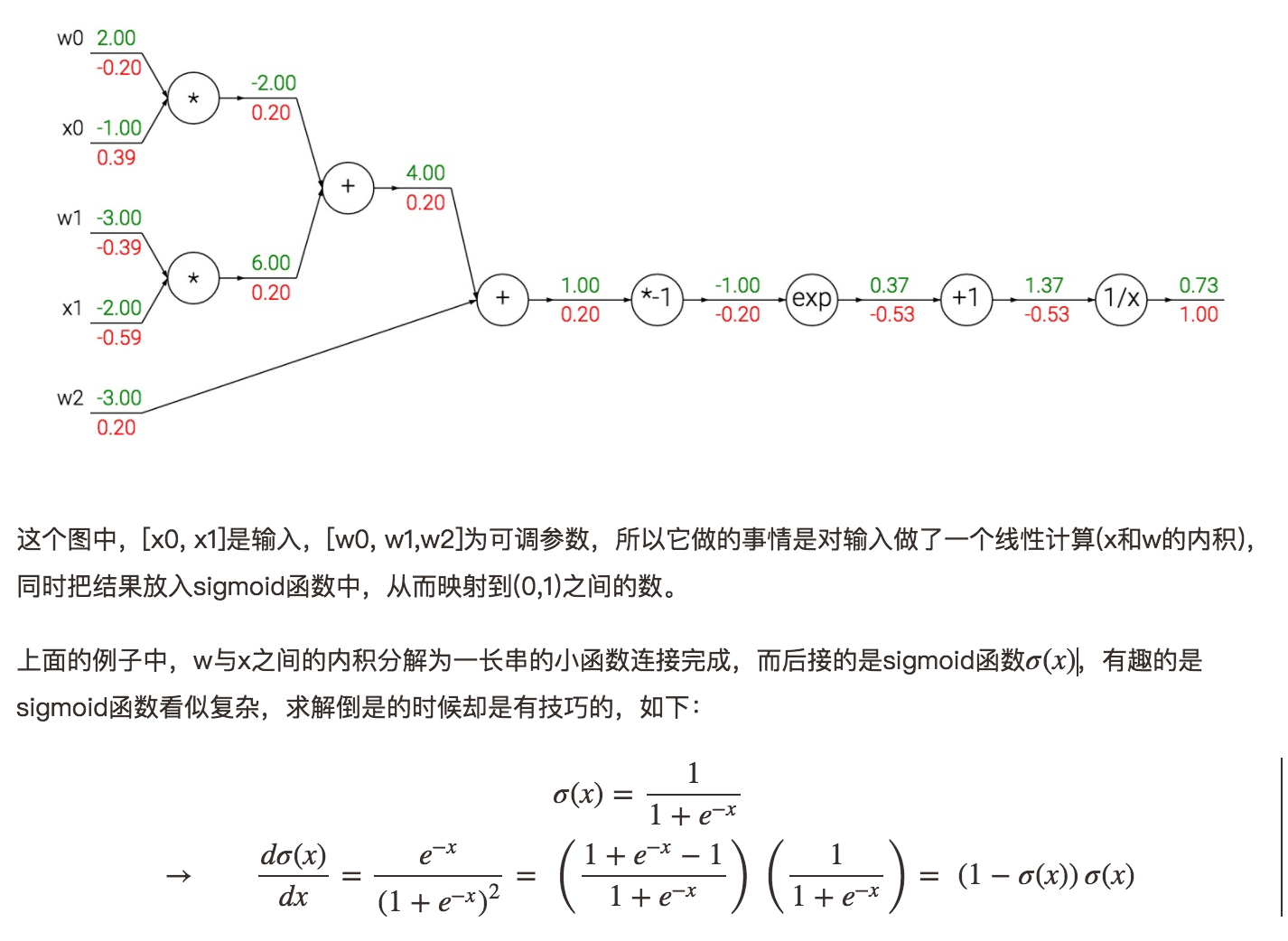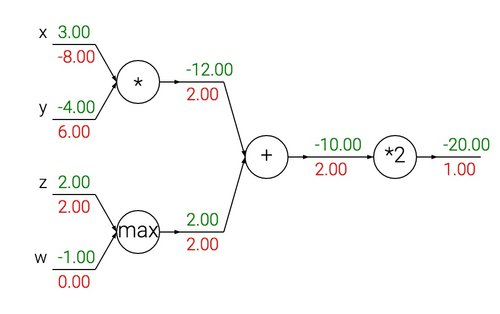# 计算机视觉之反向传播

Posted by jjx on March 1, 2017

• 大家都知道的，其实我们就是在给定的图像像素向量x和对应的函数f(x)，然后我们希望能够计算f在x上的梯度(∇f(x))
• 我们之所以想解决这个问题，是因为在神经网络中，f对应损失函数L，而输入x则对应训练样本数据和神经网络的权重W。举一个特例，损失函数可以是SVM loss function，而输入则对应样本数据(xi,yi),i=1…N和权重以及bias W,b。需要注意的一点是，在我们的场景下，通常我们认为训练数据是给定的，而权重是我们可以控制的变量。因此我们为了更新权重的等参数，使得损失函数值最小，我们通常是计算f对参数W,b的梯度。不过我们计算其在xi上的梯度有时候也是有用的，比如如果我们想做可视化以及了解神经网络在『做什么』的时候。x = -2; y = 5; z = -4

# 前向计算
q = x + y # q becomes 3
f = q * z # f becomes -12

# 类反向传播:
# 先算到了 f = q * z
dfdz = q # df/dz = q
dfdq = z # df/dq = z
# 再算到了 q = x + y
dfdx = 1.0 * dfdq # dq/dx = 1 恩，链式法则
dfdy = 1.0 * dfdq # dq/dy = 1


• 输出值
• 对应输入和输出的局部梯度

Sigmoid例子w = [2,-3,-3] # 我们随机给定一组权重
x = [-1, -2]

# 前向传播
dot = w*x + w*x + w
f = 1.0 / (1 + math.exp(-dot)) # sigmoid函数

# 反向传播经过该sigmoid神经元
ddot = (1 - f) * f # sigmoid函数偏导
dx = [w * ddot, w * ddot] # 在x这条路径上的反向传播
dw = [x * ddot, x * ddot, 1.0 * ddot] # 在w这条路径上的反向传播
# yes！就酱紫算完了！是不是很简单？


$f(x,y)=\frac{x+\sigma(y)}{\sigma(x)+(x+y)^2}$

x = 3 # 例子
y = -4

# 前向传播
sigy = 1.0 / (1 + math.exp(-y)) # 单值上的sigmoid函数
num = x + sigy
sigx = 1.0 / (1 + math.exp(-x))
xpy = x + y
xpysqr = xpy**2
den = sigx + xpysqr
invden = 1.0 / den
f = num * invden # 完成！


# 局部函数表达式为 f = num * invden
dnum = invden
dinvden = num
# 局部函数表达式为 invden = 1.0 / den
dden = (-1.0 / (den**2)) * dinvden
# 局部函数表达式为 den = sigx + xpysqr
dsigx = (1) * dden
dxpysqr = (1) * dden
# 局部函数表达式为 xpysqr = xpy**2
dxpy = (2 * xpy) * dxpysqr                                        #(5)
# 局部函数表达式为 xpy = x + y
dx = (1) * dxpy
dy = (1) * dxpy
# 局部函数表达式为 sigx = 1.0 / (1 + math.exp(-x))
dx += ((1 - sigx) * sigx) * dsigx # 注意到这里用的是 += !!
# 局部函数表达式为 num = x + sigy
dx += (1) * dnum
dsigy = (1) * dnum
# 局部函数表达式为 sigy = 1.0 / (1 + math.exp(-y))
dy += ((1 - sigy) * sigy) * dsigy
# 完事！• max(取最大)门不像加法门，在反向传播计算中，它只会把传回的梯度回传给一条输入路径。因为max(x,y)只对x和y中较大的那个数，偏导为+1.0，而另一个数上的偏导是0。
• 乘法门就更好理解了，因为xy对x的偏导为y，而对y的偏导为x，因此在上图中x的梯度是-8.0，即-4.02.0

# 前向传播运算
W = np.random.randn(5, 10)
X = np.random.randn(10, 3)
D = W.dot(X)

# 假如我们现在已经拿到了回传到D上的梯度dD
dD = np.random.randn(*D.shape) # 和D同维度
dW = dD.dot(X.T) #.T 操作计算转置， dW为W路径上的梯度
dX = W.T.dot(dD)  #dX为X路径上的梯度